### Centers of Equilateral Triangle

Illustration of an equilateral triangle that shows both the centroid (where the medians of the sides…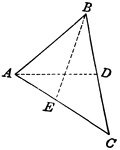### Triangle with Medians

Triangle with medians drawn.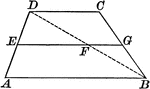### Trapezoid With Median

Illustration of a trapezoid, with the median drawn. The median of a trapezoid is parallel to the bases,…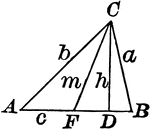### Triangle With Height and Median

Triangle with height/altitude h and median m.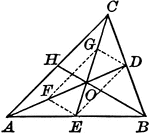### Centroid of Triangle

Illustration to show medians in a triangle. It is known as the centroid.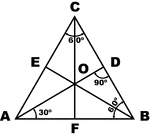### Equilateral Triangle

Sketch of equilateral triangle with bisectors, medians, and altitudes.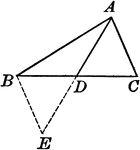### Triangle With Median Drawn

Illustration of a triangle with the median drawn.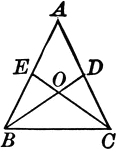### Medians Drawn Drawn to Legs of Isosceles Triangles

Illustration to show the medians drawn to the legs of an isosceles triangle.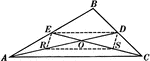### Medians In A Triangle

Illustration used to show "Any two medians of a triangle intersect each other in a trisection point…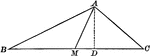### Obtuse Triangle

Obtuse triangle with altitude and one median drawn.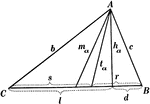### Parts Of A Triangle

Illustration used to show the various parts of a triangle: sides, angles, medians, altitudes, bisectors,…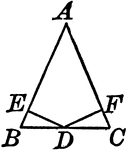### Perpendiculars Dropped From Midpoint of Base to Legs of Isosceles Triangles

Illustration to show the perpendiculars dropped from the midpoint of the base to the legs of an isosceles…